# Quadrilaterals Lesson 10 5 Identify and Classify Quadrilaterals

• Slides: 20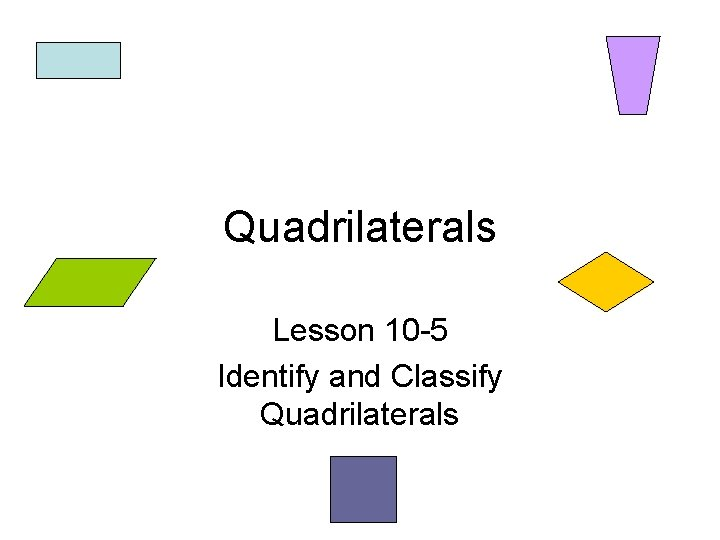Quadrilaterals Lesson 10 -5 Identify and Classify QuadrilateralsQuadrilateral • A closed figure with four sides and four angles. • Quadrilaterals are named based on their sides and angles. • The diagram shows how the quadrilaterals are related.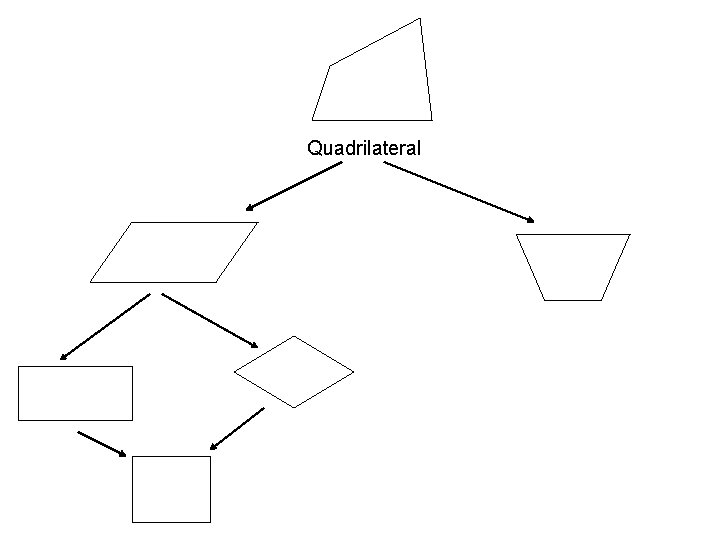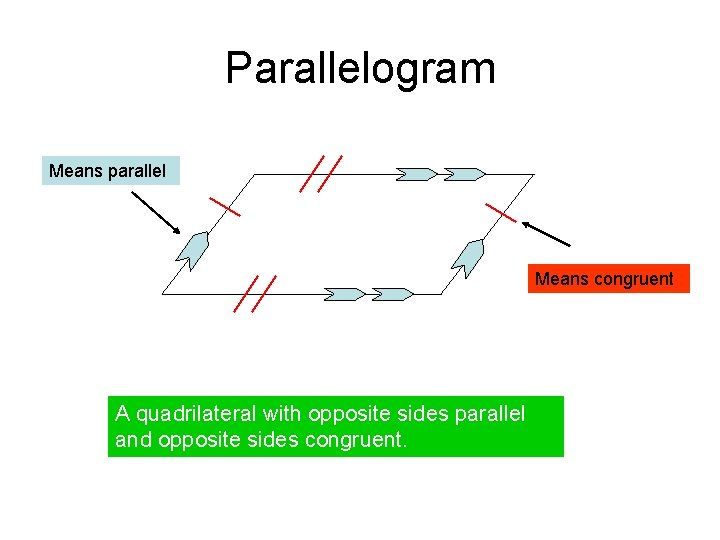Parallelogram Means parallel Means congruent A quadrilateral with opposite sides parallel and opposite sides congruent.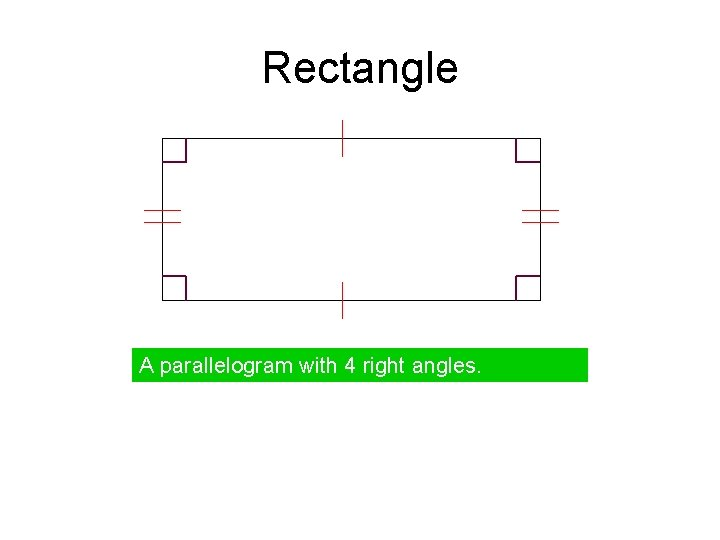Rectangle A parallelogram with 4 right angles.Rhombus A parallelogram with 4 congruent sides.Square A parallelogram with 4 right angles and 4 congruent sides.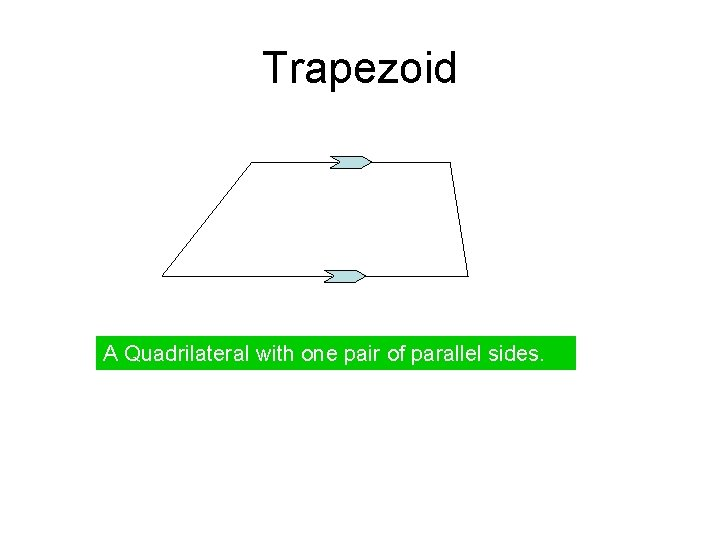Trapezoid A Quadrilateral with one pair of parallel sides.Quadrilateral Parallelogram Rhombus Rectangle Square Trapezoid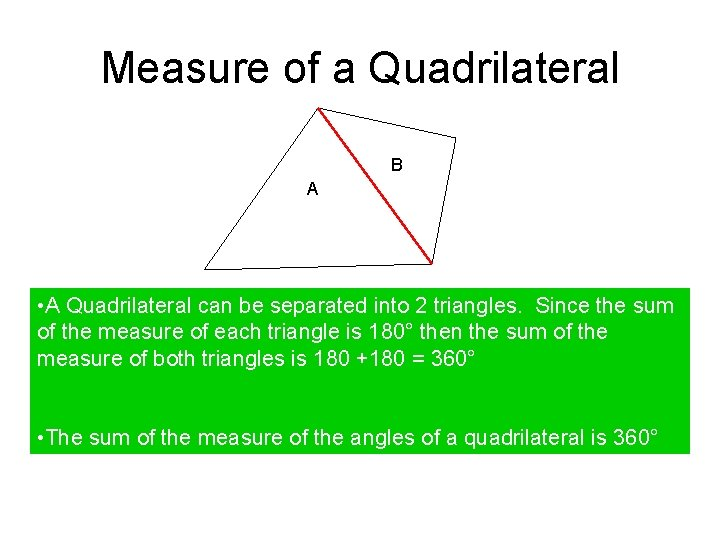Measure of a Quadrilateral B A • A Quadrilateral can be separated into 2 triangles. Since the sum of the measure of each triangle is 180° then the sum of the measure of both triangles is 180 +180 = 360° • The sum of the measure of the angles of a quadrilateral is 360°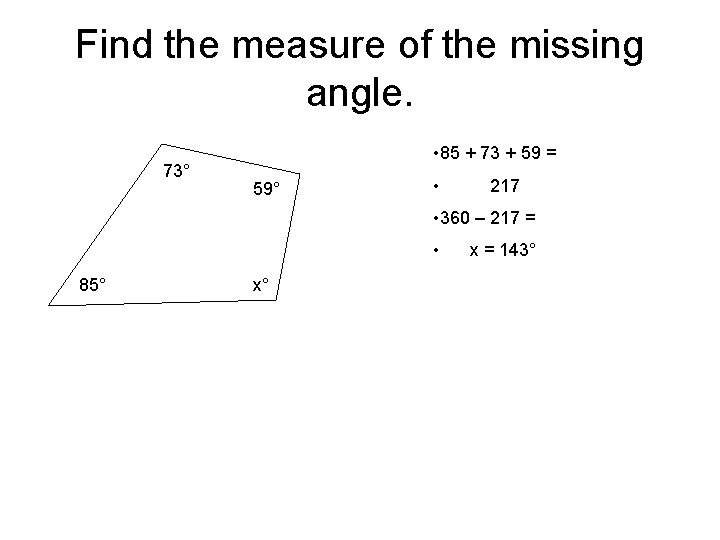Find the measure of the missing angle. 73° • 85 + 73 + 59 = 59° • 217 • 360 – 217 = • 85° x° x = 143°Concept Check • Explain why all squares are rectangles but not all rectangles are squares?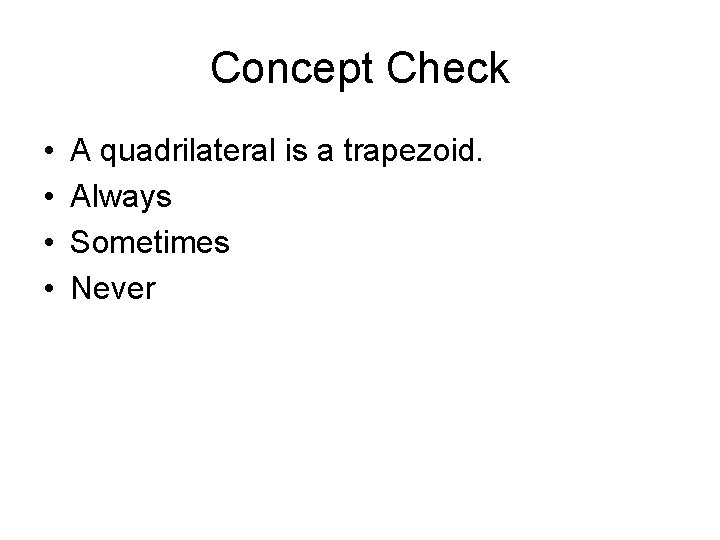Concept Check • • A quadrilateral is a trapezoid. Always Sometimes NeverConcept Check • • A quadrilateral is a trapezoid. Always Sometimes Never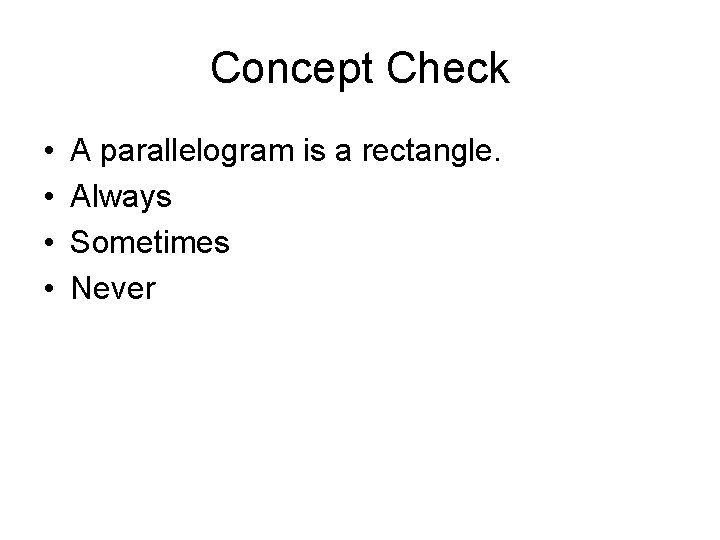Concept Check • • A parallelogram is a rectangle. Always Sometimes Never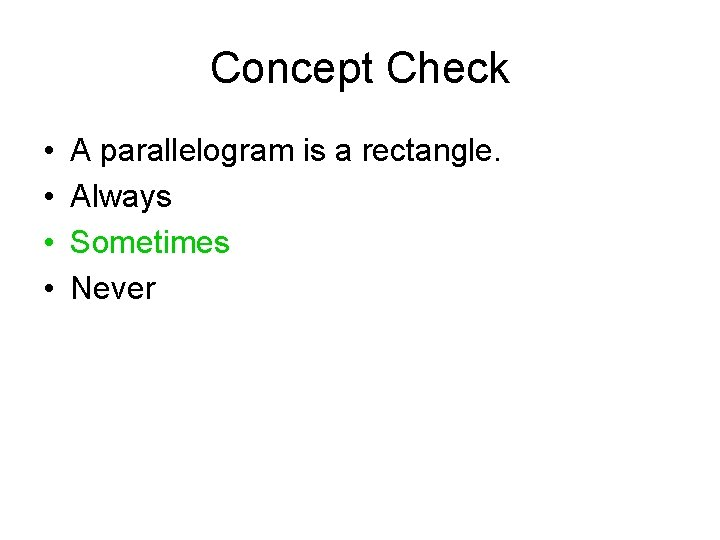Concept Check • • A parallelogram is a rectangle. Always Sometimes Never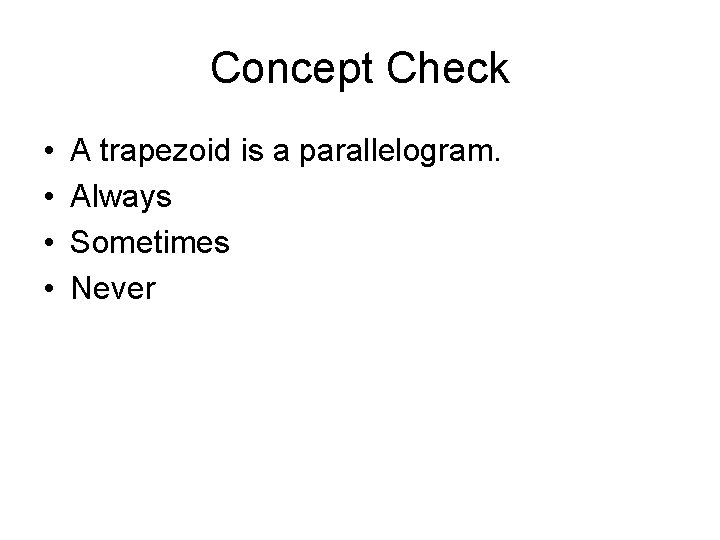Concept Check • • A trapezoid is a parallelogram. Always Sometimes Never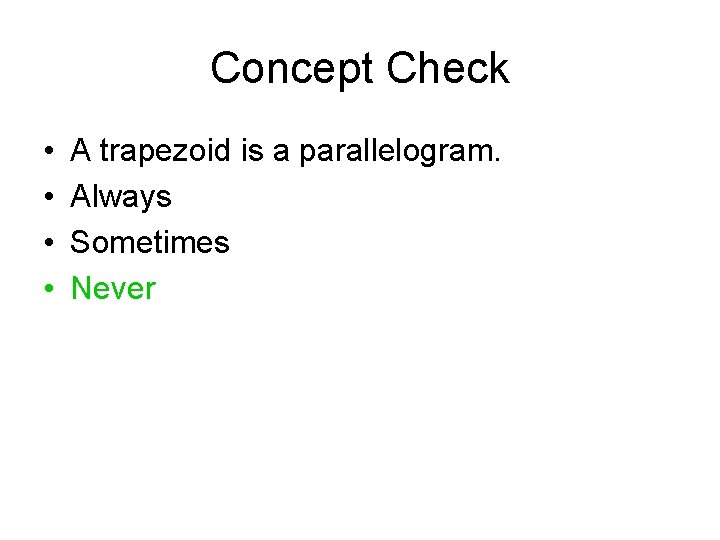Concept Check • • A trapezoid is a parallelogram. Always Sometimes NeverPractice Work • Lesson 10 -5 pg 435 - 436 • # 4 – 24 all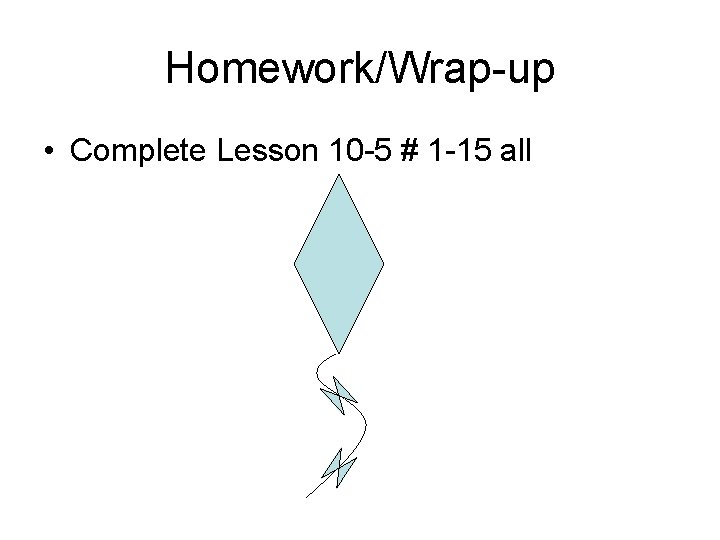Homework/Wrap-up • Complete Lesson 10 -5 # 1 -15 all